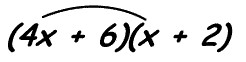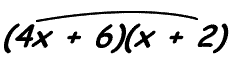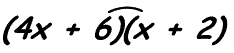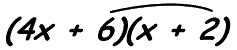# Using the FOIL Method to Simplify

You already know how to simplify an expression like $$7(4x + 3)$$, right? Just use the distributive property to multiply 7 times 4x and then 7 times 3. This gives you an answer of $$28x + 21$$. Pretty simple. But - What if you have something like this: $$(4x + 6)(x + 2)$$? That's where we use the FOIL method. FOIL means First, Outside, Inside, Last. That's not too hard to remember if you say it in your head a few times.

You use FOIL to multiply the terms inside the parenthesis in a specific order: first, outside, inside, last. Here's how to solve $$(4x + 6)(x + 2)$$:

First - multiply the first term in each set of parenthesis:$$4x * x = 4x^2$$

Outside - multiply the two terms on the outside:$$4x * 2 = 8x$$

Inside - multiply both of the inside terms:$$6 * x = 6x$$

Last - multiply the last term in each set of parenthesis:$$6 * 2 = 12$$

Now just add everything together to get $$4x^2 + 14x + 12$$. This method only works easily with two binomials. To multiply something complicated like $$(4x + 6)(5x - 3)(15 - x)$$, just do FOIL on two of the binomials and then distribute the answer onto the remaining binomial.

Here are some more examples of FOIL multiplication:

### Example:

Multiply the following: $$(6x+1)(2x+9)$$

### Solution:

Just follow the letters in FOIL:

First: $$6x*2x=12x^2$$

Outside: $$6x*9=54x$$.

Inside: $$1*2x=2x$$.

Last: $$1*9=9$$.

Sum it all up and you get: $$12x^2+56x+9$$.

### Example:

Multiply the following: $$(2x-5)(x-4)$$

### Solution:

Just follow the letters in FOIL:

First: $$2x*x=2x^2$$

Outside: $$2x*(-4)=-8x$$

Inside: $$-5*x=-5x$$

Last: $$(-5)*(-4)=20$$

Sum it all up and you get: $$(2x^2-13x+20)$$.

The FOIL method is not too difficult to learn once you remember what it stands for. Just repeat first, outside, inside, last and you'll remember it. Other than that, it's just a matter of multiplying each of those steps and adding everything together. Even if the numbers are really ugly with fractions and negative signs and such just follow the steps and the method will work.

If you have more questions about FOIL, as always feel free to ask for help on the math help message board, or check out the FOIL calculator below.